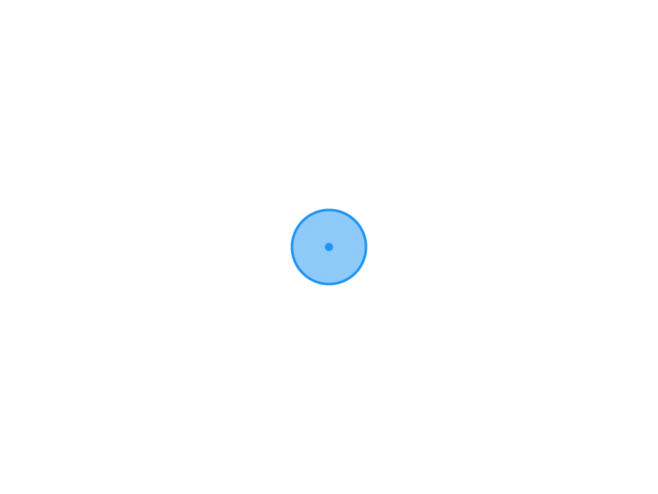TypechoJoeTheme

# 日志随记

/XG.孤梦

# C语言函数的调用-学习十七2022-02-11
/
0 评论
/
655 阅读
/
1392 个字
/

02/11

## 函数调用的形式

• 一般形式为：

• ` 函数名` `(实参表列)`
• 如果是调用无参函数，则“实参表列”可以没有，但括号不能省略

• `max();`
• 如果实参表列包含多个实参，则各参数间用逗号隔开

• `max(a,b);`
• 按函数调用在程序中出现的形式和位置来分，
可以有以下3种函数调用方式:

• 1.函数调用语句

• 把函数调用单独作为一个语句。如：` printf_star();`
• 这时不要求函数带回值，只要求函数完成一定的操作。
• 2.函数表达式

• 函数调用出现在另一个表达式中。如：`c=max(a,b);`
• 这时要求函数带回一个确定的值。
• 3.函数参数

• 函数调用作为另一函数调用时的实参。如：`m=max(a，max(b，c));`
• 其中 `max(b，c)` 是一次函数调用，它的值作为max另一次调用的实参。

## 函数调用时的数据传递

• 调用有参函数时，主调函数和被调用函数之间有数据传递关系。
• 定义函数时函数名后面的变量名称为“形式参数”(简称“形参”)。
• 主调函数中调用一个函数时，函数名后面参数称为“实际参数”（简称“实参”)。
• 在调用函数过程中，系统会把实参的值传递给被调用函数的形参
该值在函数调用期间有效，可以参加被调函数中的运算。

## 函数调用的过程

• 在定义函数中指定的形参，在未出现函数调用时，它们并不占内存中的存储单元
发生函数调用时，函数的形参被临时分配内存单元
• 实参与形参的类型应相同或赋值兼容
• C语言规定，实参变量对形参变量的数据传递是“值传递”，即单向传递，只由实参传给形参，而不能由形参传回来给实参
• 在内存中，实参单元与形参单元是不同的单元

## 函数的返回值

• 通常，希望通过函数调用使主调函数能得到一个确定的值，这就是函数值(函数的返回值)。
• 函数的返回值是通过函数中的return语句获得的。
• 一个函数中可以有一个以上的return语句，执行到哪一个return语句，哪一个就起作用
• return语句

• 一般形式为：`return` `表达式;` 或者为：`return` `(表达式);`
• 例如：`return(z);` 或者：`return Z;`• 如果被调用函数中没有return语句，说明程序员没有希望得到函数值。
• 但实际上，函数并不是不带回值，而只是不带回有用的值，带回的是一个不确定的值
• 为了明确表示“不带回值”，可以用“void”定义函数“无类型”(或称 “空类型”)，此时函数中不得有return语句。
• 例如:

• `void printstar(){ ... }`
• 函数值的类型和函数定义中函数的类型应保持一致
如果函数值的类型和return语句中表达式的值不一致，以函数类型为准
• 凡不加类型说明的函数，自动按整型处理

## 被调用函数的声明和函数原型声明

• 在一个函数中调用另一个函数需要具备如下条件:

• 1.被调用函数必须是已经定义的函数(是库函数或用户自己定义的函数)。
• 2.如果使用库函数，应该在本文件开头加相应的#include指令
• 3.如果使用自己定义的函数，而该函数的位置在调用它的函数后面，应该在前面声明

### 注意:

• “定义”是对函数功能的确立，包括指定函数名，函数类型、形参及类型，语句等，是一个完整、独立的函数单位。
• “声明”是把函数的名字、函数类型和形参的类型、个数以及顺序通知编译系统，以便在调用该函数时系统按此进打对照检查。
• 函数原型声明(函数声明是使用函数原型来声明的)。

• `函数类型` `函数名(参数类型1 参数名1,数类型2 参数名2...);`
• `函数类型` `函数名(参数类型1,参数类型2......);`
• 例如：

• `float add(float x, float y);`
• `float add(float, float);`
• 如果在函数调用之前，没有对函数作说明，则编译系统会把第一次遇到的该函数形式 (函数定义或函数调用)作为函数的声明，并将函数类型默认为int型
• 如果被调用的函数类型为整型，则允许在调用函数前不做声明
• 如果被调用函数的定义出现在主调用函数之前，可不必声明。

• 例如
``````#include <stdio.h>

// 主调用函数之前定义函数
float add(float x, float y) {
float z;
z = x + y;
return z;
}

main() {
float a, b, c;
printf("请输入两个实数：\n");

scanf_s("%f %f", &a, &b);

printf("结果：%f\n", c);
}``````
• 如果已经在文件的开头( 所有函数之前)，对本文中所调用的函数进行了声明，则在各函数中不必对其所调
用的函数再作声明。

• 例如
``````#include <stdio.h>

float add(float x, float y); //所有函数之前声明

main() {
float a, b, c;
printf("请输入两个实数：\n");

scanf_s("%f %f", &a, &b);

printf("结果：%f\n", c);
}

float add(float x, float y) {
float z;
z = x + y;
return z;
}``````

#### 例子

``````#include <stdio.h>

main() {
int max(float x, float y);

float a,b;
int c;
printf("请输入两个实数：\n");
scanf_s("%f %f", &a, &b);
c = max(a, b);

printf("结果：%d\n", c);
}

int max(float x, float y) {
float z;
if (x > y) { z = x; }
else { z = y; }
return z;
}````````````#include <stdio.h>

main() {

float a, b,c;
printf("请输入两个实数：\n");

scanf_s("%f %f", &a, &b);

printf("结果：%f\n",c );
}

float add(float x, float y) {
float z;
z = x + y;
return z;
}````````````#include <stdio.h>
#include <math.h>

main() {
int leaps(int x);
int m, n = 0;
for (m = 101; m <200; m=m+2) //偶数不可能是素数
if (leaps(m) == 1) {
n++;
printf("%d, ", m);
if (n % 5 == 0) {
printf("\n");
}
}
}

int leaps(int x) {
int i, j, leap;
j = sqrt(x); //减少计算次数
for (i = 2; i <= j; i++)
if (x % i == 0) {
leap = 0;
break;
}
else {
leap = 1;
}
return leap;
}``````https://www.xggm.top/archives/440.html（转载时请注明本文出处及文章链接）XG.孤梦博 主大佬

51 文章数
102 评论量

X